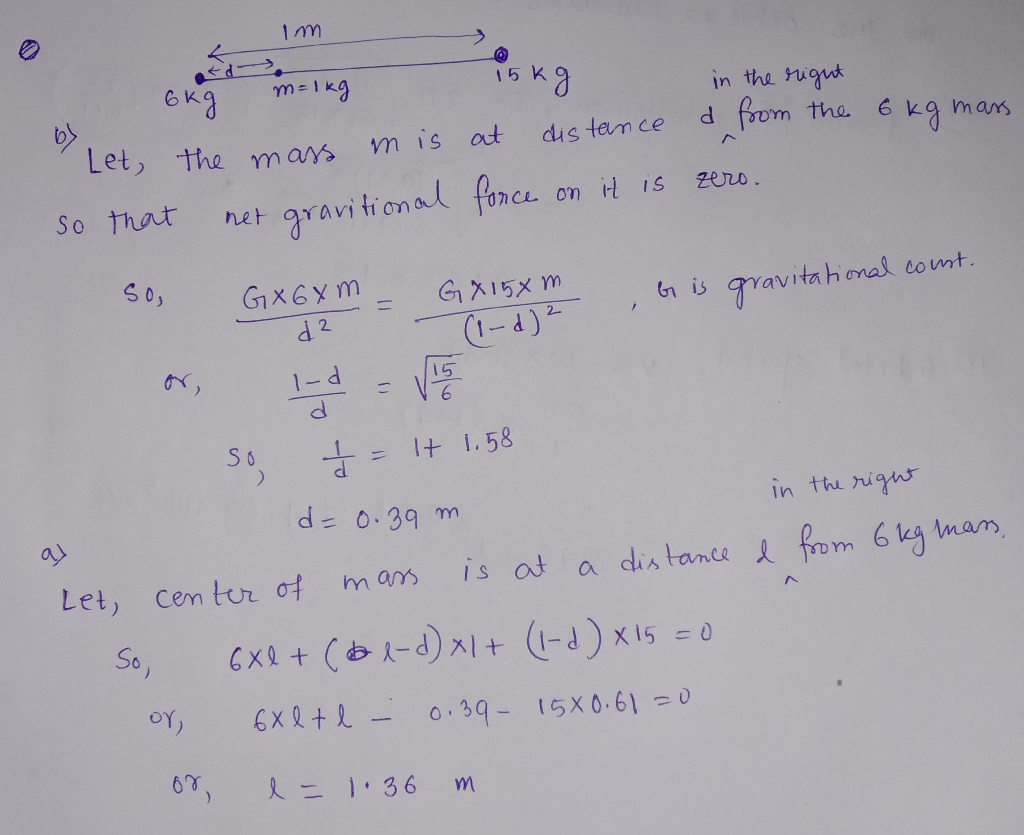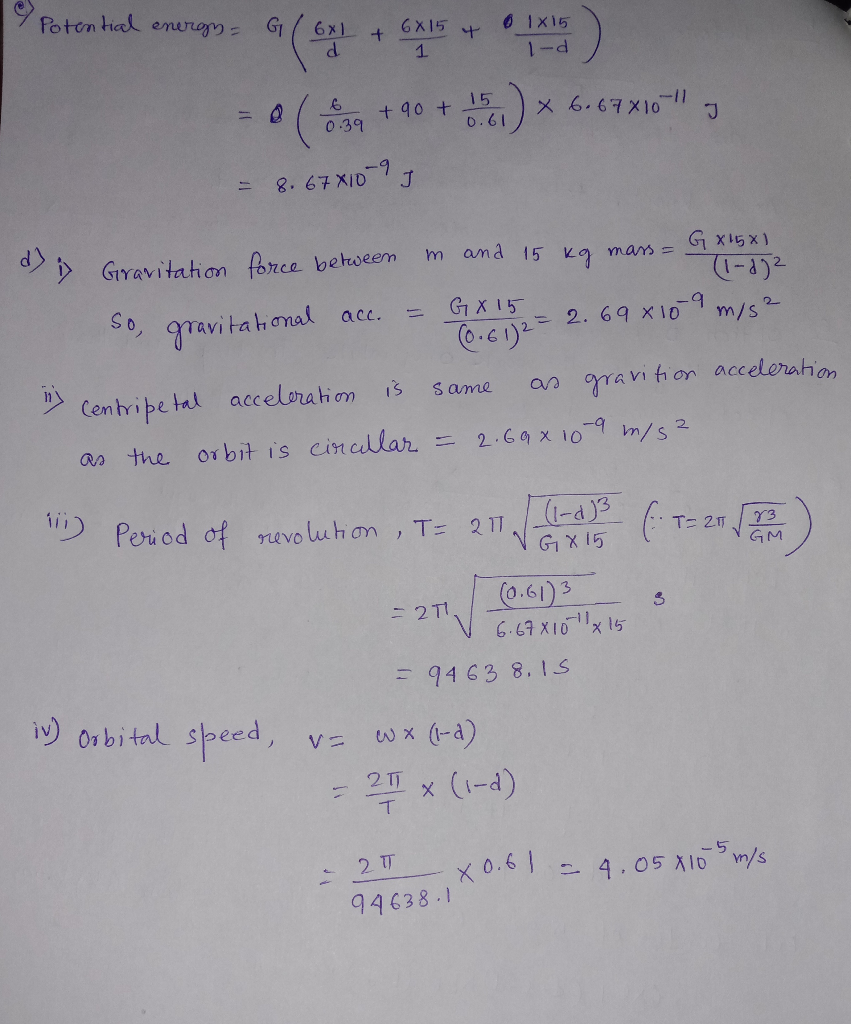In: Physics

# A 6.00-kg point mass and a 15.0-kg point mass are held in place 1.00 m apart....

A 6.00-kg point mass and a 15.0-kg point mass are held in place 1.00 m apart. A point particle of mass m = 1.00 kg is released from a point between the two masses such that the net gravitational force on this particle is zero. Call the line connecting all the masses x-axis.
a. Find the center of mass of this system.
b. Find the exact position of this point mass.
c. Calculate potential energy of this system.
d. If the 6.00-kg mass is then removed and m is allowed to orbit the 15.0-kg mass, calculate (i) the gravitational acceleration due to the 15.0-kg mass at the location of m, (ii) the centripetal acceleration of m (hint: how does the centripetal acceleration relate to the gravitational acceleration?), (iii) the period of its revolution around the 15.0-kg mass, assuming that it stays at the same distance away from the 15.0-kg mass, and (iv) the orbital speed of m around the 15.0-kg mass.

## Solutions

##### Expert Solution## Related Solutions

##### (a) A light, rigid rod of length 1.00 m joins two particles, with masses m-4.00 kg...
(a) A light, rigid rod of length 1.00 m joins two particles, with masses m1 = 4.00 kg and m2 = 3.00 kg, at its ends. The combination rotates in the xy-plane about a pivot through the center of the rod (see figure below . Determine the angular momentum of the system about the origin when the speed of each particle is 4.40 m/s. (Enter the magnitude to at least two decimal places in kg·m2/s. (b) What I? What would be...
##### A disk with mass m = 11.8 kg and radius R = 0.31 m begins at...
A disk with mass m = 11.8 kg and radius R = 0.31 m begins at rest and accelerates uniformly for t = 17.2 s, to a final angular speed of ω = 31 rad/s. 1.What is the angular acceleration of the disk? 2. What is the angular displacement over the 17.2 s? 3. What is the moment of inertia of the disk? 4. What is the change in rotational energy of the disk?
##### At t = 0, an electron (mass = 9.11 ×10-31 kg) is released from rest 1.00...
At t = 0, an electron (mass = 9.11 ×10-31 kg) is released from rest 1.00 meter above the center of a charged, circular ring, which has a total charge of –3.00 nC. The diameter of the ring is 2.00 meters and the plane of the ring is oriented horizontally. What are the magnitude and direction of the acceleration of the electron, just after it is released?
##### A simple pendulum with mass m = 1.6 kg and length L = 2.79 m hangs...
A simple pendulum with mass m = 1.6 kg and length L = 2.79 m hangs from the ceiling. It is pulled back to an small angle of θ = 10.7° from the vertical and released at t = 0. A)What is the period of oscillation? B)What is the magnitude of the force on the pendulum bob perpendicular to the string at t=0? C)What is the maximum speed of the pendulum? D)What is the angular displacement at t = 3.62...
##### The person’s mass, m= 68.1 kg, the unknown drag coefficient is, c , with units kg/s,...
The person’s mass, m= 68.1 kg, the unknown drag coefficient is, c , with units kg/s, and the local acceleration of gravity is g= 9.80 m/s2 . Our model for the person’s velocity vs time gave us a linear differential equation whose analytical solution was the following: V(t) = g*m/c*[1-exp(-c/m*t)]. Your job is to find “c”. You need to use the MATLAB “help” documentation to find the nonlinear curve fitting function and syntax for how to use it. Hint you...
##### Three asteroids of identical mass (M = 1.03×1012 kg) are orbiting their common center of mass...
Three asteroids of identical mass (M = 1.03×1012 kg) are orbiting their common center of mass in a perfect circle of radius R=75.8 km. a. What is the period of orbit of one of these asteroids? You are standing on one of the asteroids. You are standing on the side of the asteroid which faces out from the circle. Your goal is to jump up off the asteroid and escape the entire three asteroid system. The radius of the asteroid...
##### A +6.00 -μC point charge is moving at a constant 8.00×106 m/s in the + y-direction,...
A +6.00 -μC point charge is moving at a constant 8.00×106 m/s in the + y-direction, relative to a reference frame. At the instant when the point charge is at the origin of this reference frame, what is the magnetic-field vector it produces at the following points. Part A: x = +.5 m, y = 0 m, z = 0 m Part B: x = 0 m, y = -.5 m, z = 0 m Part C: x = 0...
##### A car with a mass of 1000 kg and a speed of v1 = 20.0 m/s...
A car with a mass of 1000 kg and a speed of v1 = 20.0 m/s approaches an intersection, as shown in the figure(Figure 1). A 1230 kg minivan traveling at v2 is heading for the same intersection. The car and minivan collide and stick together. The direction of the wreckage after the collision is θ = 44.0 ∘ above the x-axis. You may want to review(Pages 272 - 277). Part A: Find the initial speed of the minivan, assuming...
##### As shown in the figure, a conducting rod with a linear mass density of 0.0395 kg/m...
As shown in the figure, a conducting rod with a linear mass density of 0.0395 kg/m is suspended by two flexible wires of negligible mass in a uniform magnetic field directed into the page. A power supply is used to send a current through the rod such that the tension in the support wires is zero. (a) If the magnitude of the magnetic field is 3.70 T, determine the current in the conducting rod. (b) Determine the direction of the...